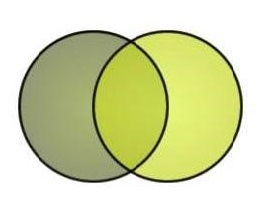# wavelength

n=5

a: wavelength ~
b: circumference of the tire

What:

"Think in terms of your car. The frequency is like how many times a minute your tires turn. The wavelength is like the circumference of the tire. If you know the size of the wheel and how fast it's spinning, you can figure out how fast the car is moving with a little math. "

Useful?
Writer: Wazowsk
LCC:
Date: Jan 14 2015 6:44 PM

a: Wavelength ~
b: the distance between two wave crests on the ocean

What:

"Radio energy moves in waves, and each radio wave has not only a frequency but also a wavelength. The wavelength is like the distance between two wave crests on the ocean. With radio energy, the wavelength of a radio wave multiplied by its frequency is equal to the speed of light: 3x108
meters per second (roughlyequal to 186,000 miles per second). The size of waves for each of the unlicensed bands is presented in Table 2.3"

Useful?
Writer: Simson Garfinkel Henry Holtzman
LCC:
Where:
Date: Jan 14 2015 6:47 PM

a: wavelength ~
b: distance of the peoples' steps

What:

"Imagine a short person and a tall person walking side by side. The short person takes much smaller steps while the tall person takes larger steps. In order for the two people to walk at the same speed, the shorter person must walk faster and the tall person must walk slower. This is much like the relationship between the wavelength and frequency of light. The wavelength is like the distance of the peoples' steps and the frequency is how fast each step is taken. All light travels at the same speed. The wavelength of light is the distance spanned by one cycle of motion. The larger this distance, the slower the frequency (how quickly the motion oscillates) must be in order to maintain the speed of light. Smaller wavelengths must have faster frequencies to maintain the speed of light. This explains why wavelength is inversely proportional to frequency."

Useful?
Writer: Not Stated
LCC:
Where:
Date: Jan 14 2015 6:52 PM

a: wavelength ~
b: distance between two adjacent peaks

What:

"Wavelength is like the distance between two adjacent peaks or two adjacent valleys. Stated another way, wavelength is the time required to complete one full cycle of the wave."

Useful?
Writer: Thomas Zesiger
LCC:
Where:
Date: Jan 14 2015 6:54 PM

a: wavelength ~
b: crest to crest and trough to trough

What:

Think of ocean waves when learning this, they are good proxy for electromagnetic radiation.

Useful?
Writer: The One True Bobo
LCC: